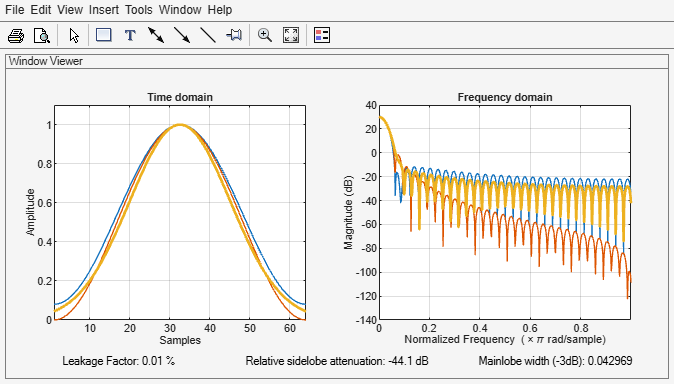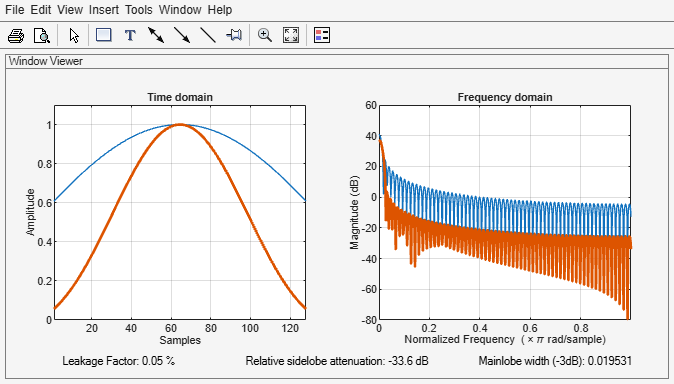# WVTool

Open Window Visualization Tool

## Description

Window Visualization Tool is an interactive tool that enables you to visualize time and frequency domain plots of the window vector. You can generate window vectors for a number of common window functions using the Signal Processing Toolbox™ software. See `window` for a list of supported window functions.

Note

A related tool, Window Designer, is available for designing and analyzing windows.

## Open the WVTool

WVTool can be opened programmatically using one of the methods described in Programmatic Use.

## Examples

expand all

Use `wvtool` to display and compare 64-point Hamming, Hann, and Gaussian windows.

`wvtool(hamming(64),hann(64),gausswin(64))`Compare 128-point Kaiser windows with different values of $\beta$.

`wvtool(kaiser(128,1.5),kaiser(128,4.5))`## Programmatic Use

expand all

`wvtool(WindowVector)` opens the Window Visualization Tool (WVTool) with time and frequency domain plots of the window vector specified in `WindowVector`. `WindowVector` must be a real-valued row or column vector. By default, the frequency domain plot is the magnitude squared of the Fourier transform of the window vector in decibels (dB). You can generate window vectors for a number of common window functions using the Signal Processing Toolbox software. See `window` for a list of supported window functions.

`wvtool(WindowVector1,...,WindowVectorN)` opens WVTool with time and frequency domain plots of the window vectors specified in `WindowVector1`, …, `WindowVectorN`.

`H = wvtool(...)` returns the figure handle, `H`.

expand all